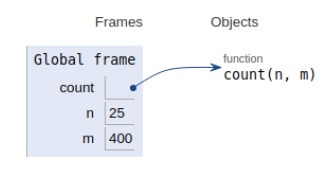# Python Program for Number of elements with odd factors in the given range

PythonServer Side ProgrammingProgramming

#### Beyond Basic Programming - Intermediate Python

Most Popular

36 Lectures 3 hours

#### Practical Machine Learning using Python

Best Seller

91 Lectures 23.5 hours

#### Practical Data Science using Python

22 Lectures 6 hours

In this article, we will learn about the solution to the problem statement given below.

Problem statement − We are given a range, we need to find the number of odd factors in the range.

## Approach

As we all know that all perfect squares have an odd number of factors in a range. So here we will compute a number of perfect squares.

As m and n both are inclusive, so to avoid error in case of n being a perfect square we take n-1 in the formulae.

Now let’s see the implementation below−

## Example

Live Demo

# count function
def count(n, m):
return int(m**0.5) - int((n-1)**0.5)
# main
n = 25
m = 400
print("Number of odd squares are: ", count(n, m))

## Output

Number of odd squares are: 16All the variables and functions are declared in the global scope as shown in the figure above.

## Conclusion

In this article, we have learned how we can find the number of elements with odd factors in a given range.